# VBA Loop

It is a programming construct that allows you to execute a block of code multiple times.Reviewed by

Christopher Ballesteros

Expertise: Consulting | Private Equity

Updated:

January 24, 2023

Microsoft Visual Basic for Applications (VBA) is a programming language used to create custom functions and automate processes in Microsoft Office applications, such as Excel, Word, and PowerPointA loop is a programming construct that allows you to execute a code block multiple times. Loops are useful for tasks that need to be repeated, such as processing a large number of data records or performing a complex calculation multiple times. There are several types of loops in VBA, including

1. For loop
2. For Each loop
3. Do While loop
4. Do Until loop

Each loop mentioned above can be used while building loops which essentially consolidate the code for you and even makes it easier for a third person to read the code.

In this article, we will see all the different loops that you can use in Excel, along with different scenarios where you use them.

## How to write a VBA Loop

Once you have set up the initial code, there will always be scenarios where you need to iterate multiple times over a range of cells or a data source.

In such cases, you can write the syntax for either of the four loops according to the scenario you are building for the loop to work in.To write a loop in VBA code, you can use one of the four loop constructs: For, For Each, Do While, or Do Until. Here are some examples of how to use each type of loop in VBA:

1. For loop:

A For loop in VBA allows you to execute a block of code a specific number of times.

A starting value defines it, an ending value, and the step value increments a step value and the loop counter on each iteration of the loop.

For i = 1 to 10

' code to be executed

Next i

This loop will execute the code block ten times, with the variable "i" serving as the loop counter. The loop counter starts at 1 and is incremented by 1 on each iteration of the loop until it reaches 10.

2. For Each loop:

A For Each loop in VBA allows you to iterate over a collection of objects, such as the cells in a range of elements in an array.

It is defined by a loop variable representing each object in turn and a collection of objects to iterate.

For Each cell In Range("A1:A10")

' code to be executed

Next cell

This loop will iterate over the cells in the range A1:A10, with the variable "cell" representing each cell. The loop will execute the code block for each cell in the range.

3. Do While loop:

A Do While loop in VBA allows you to execute a code block if a certain condition is true. A condition defined is checked at the beginning of each loop iteration.

Do While condition

' code to be executed

Loop

4. Do Until loop:

A Do Until loop in VBA allows you to execute a code block until a certain condition is true. A condition defined is checked at the beginning of each loop iteration.

Do Until condition

' code to be executed

Loop

This loop is similar to a Do While loop, but it executes the code block until the specified condition is true rather than while it is true.

It's important to include a way to exit the loop, either by modifying the loop condition or using a control statement, such as Exit For or Exit Do, to prevent the loop from running indefinitely.

Next, we will see each of these loops individually and how you can use them in a VBA code.

## NOTE

Loops are used to iterate over the ‘n’ number of rows or columns to check if the data in those specific cells meet the criteria.Begin your journey into Excel modeling with our free Excel Modeling Crash Course.

## For Loop

The For Loop is one of the most important loops in VBA that allows you to execute a block of code ‘n’ several times. So let's say that you need to perform a specific task 10 times; for loop is your go-to tool.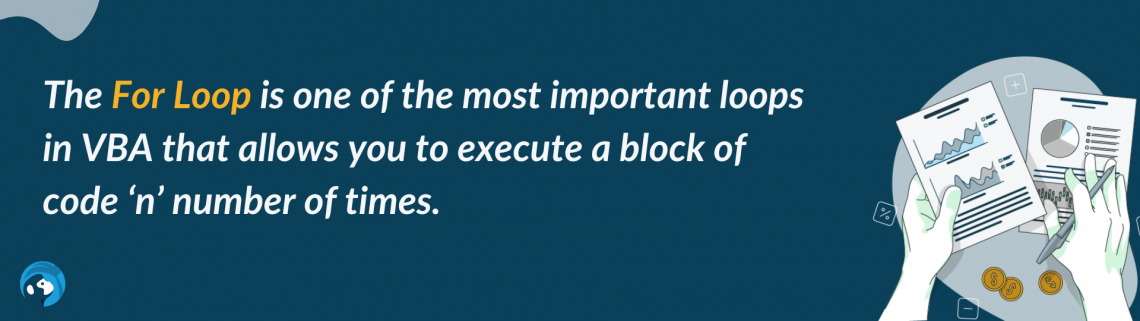We deduce here that in For loops, it is essential to know the number of iterations for the loop to work.

Suppose that if we have data only till the 10th row and set the iterations till 15, the code will still run through all the fifteen rows and perform the specified operation.

Here’s an example of how to use the loop to iterate over a range of cells and perform an operation on each cell. Suppose that you have the data illustrated below: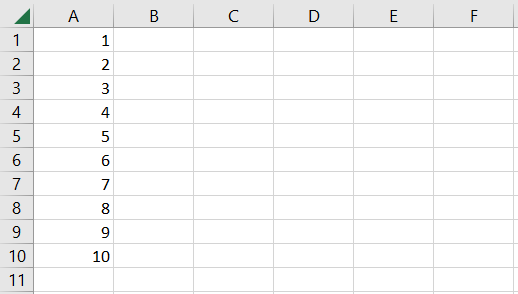We will use the code below by creating a new module and pressing the F5 key in the VBA project window.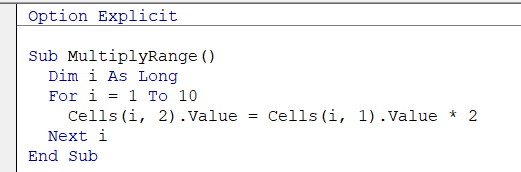Once the code finishes running, you will find that all the cells adjacent to non-empty cells in column A return some numbers. As specified in the code, whatever values are returned in column B result from cell value in column A x 2.

Thus the final result is equal to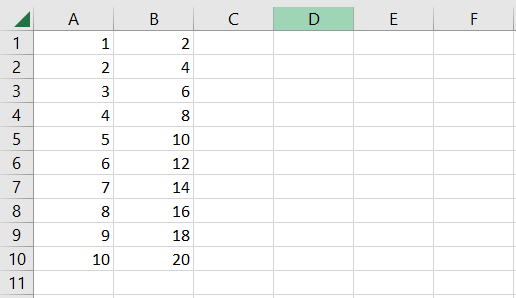If we had input the value of i = 1 to 5, then the code would have run only till the fifth row, and the rest of the cells would have been ignored by the code.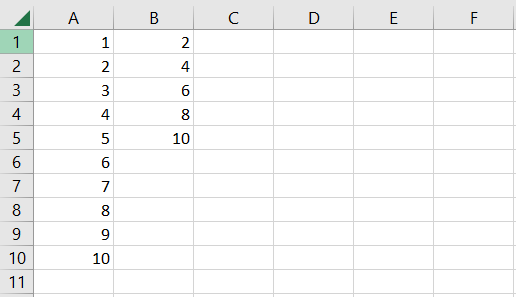The data here is limited; however, there can be thousands of rows of data in a real-life scenario. Therefore, we can only expect to write a new code that sometimes accommodates the given data.

Instead, we can use the last row/last column formula that automatically assumes the given dataset's endpoints.

You can use the below snippet of code to find the last row of the data automatically worksheets containing variable rows of the dataset and automatically loop through it.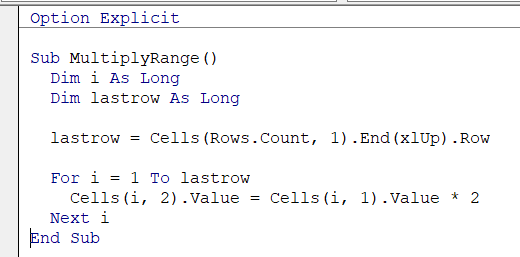This will iterate through all the rows up to the point where the last row of data lies.

## NOTE

The For Loops are constructed based on the assumption that we know how often the loop needs to be iterated through a given data.

## For Each Loop

As stated in the earlier section, a For Each Loop iterates over a collection of objects, such as cells in an array or the elements in an array.The For Each Loop is limited to the collection of objects in an array and does not go beyond that collection.

For example, if you have three elements as, apples, mangoes, and pineapple, in a collection, then the loop will end once it goes through those elements.

Until and unless you add a fourth item to the collection, the For Each Loop will act on only those three items and return the result accordingly.

Here’s an example of how to use the loop to iterate over a range of cells and perform an operation on each cell. For example, suppose you have the numbers in the range A1:A10 as illustrated below: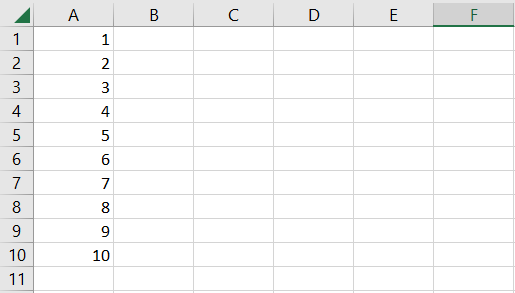Let’s say we want to multiply each number in the range A1:A10 by 2. We will use the VBA code as illustrated below: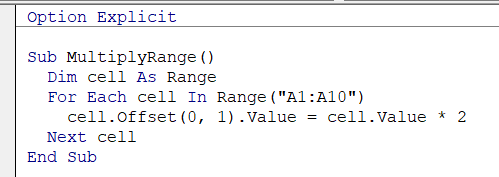This subroutine multiplies the value of each cell in the range A1:A10 by 2.

The For Each loop iterates over the cells in the range A1:A10 and executes the code block for each cell in the range, which multiplies the cell's value by 2 and stores the result in the cell adjacent to column A, i.e., in column B.

You can press the F5 key or even create a button to run the macro, which ultimately gives the result as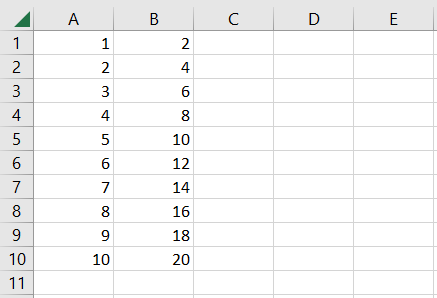As you can see, whatever number we had in column A gets multiplied by 2. For example, the following result is returned in the adjacent cell of column B.

## NOTE

The For Each loop iterates over a collection of ‘known’ items. Once the list of elements or items ends, the loop also ends.

## Do While Loop

To understand Do while loops, let’s take an example of rolling a die. As a rule for one of the board games Ludo, you need six on the dice, or else you cannot exit the safehouse.You will need to keep rolling the dice if you do not get a six. The Do While loop works similarly where the loop keeps repeating as long as a certain condition is TRUE, not getting a six on the face of the dice.

Let’s see an example of how to use the loop to iterate over a range of cells and perform an operation on each cell until a certain condition is met: Suppose that you have the data as illustrated below:Here, we will use the below snippet of code which checks whether the cells in column A are empty.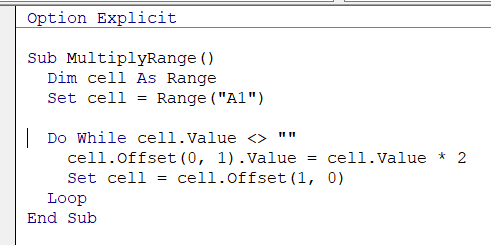If the cell is not empty, it goes ahead and performs a multiplication, i.e., A1 x 2, and returns the result in column B.

Initially, we set the counter at cell A1; however, we add another line, i.e., cell = cell.Offset(1,0) accepts the next cell for the loop. The cells go in order A1, A2, A3… till there is an empty cell to break the loop, and the subroutine ends.

The final result you will get is: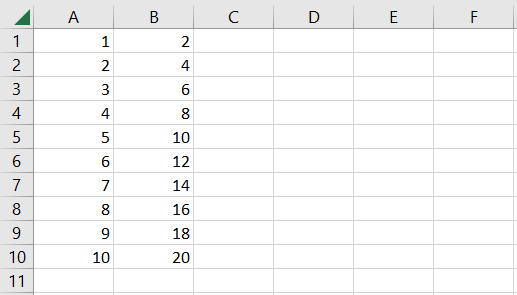This is the simplest example of using the Do While loop in VBA. You can build even the most complex Excel models, and we assure you it will work.

## NOTE

The Do While loop iterates when a certain condition is not met. Once the condition is fulfilled, the loop ends.

## Do Until Loop

The Do Until loop seems similar; there is a fine line between the Do While and Do Until loops. Earlier, we said the do-while loop keeps on iterating if a certain condition is not TRUE.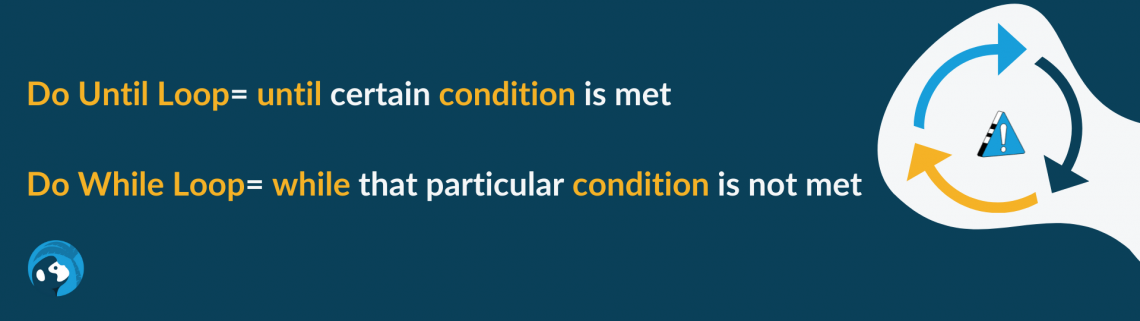We took the example of a dice where we assumed the person would keep rolling the dice if they did not get a six. On the other hand, the do until loop works ‘until’ we get a six on the dice.

For this specific example, the condition changed from not equal to (<>) six for do-while loops to equal (=) six for do-until loops.

We will see how to use the loop to iterate over a range of cells and perform an operation on each cell until a certain condition is met. For example, suppose that you have the data as illustrated below:We have a similar snippet of code where the major change is the ‘Do Until cell.Value = “”’. This will ask the code to iterate until it finally finds an empty cell.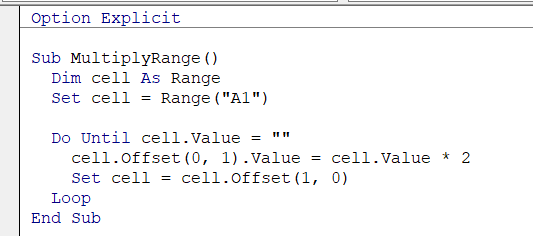Once you run the code using the F5 key on the keyboard or using a VBA button, you will get the result:It doesn’t matter what loop you use for the code; we assure you that it will only make your life easier than not using the loops in the VBA code.

## NOTE

The Do Until loop iterates ‘until’ a certain condition is met. If the condition is not fulfilled, the loop keeps iterating through the given dataset, checking for a value that fulfills the condition.

## Key Takeaways

• VBA is a programming language you can use to automate repetitive tasks in different Microsoft software.
• A macro is a series of instructions or commands written using VBA that are stored in a computer program and can be executed automatically.
• There are four different types of loops that you can use: for loops, for each loop, do until loop, and do while loop.
• The For loop will iterate through a block of code ‘n’ several times. For example, going through rows 1 to 10. You can even ‘step’ that lets you jump every second or the third row in the iteration, i.e., 1, 3, 5, 7, 9, etc.
• Each loop iterates through the entire collection of elements in a given array. The loop ends if the code block is run on all the elements.
• The Do While code loops through a code block once a certain condition is fulfilled.
• The Do Until loop will iterate a code block until a certain condition is fulfilled.

Researched and Authored by Akash Bagul LinkedIn

Reviewed & Edited by Ankit SinhaLinkedIn